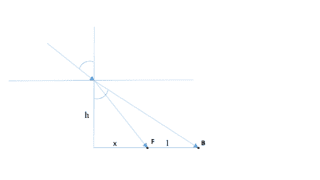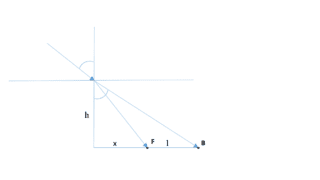# Snells law and depth of water

## Homework Statement

The light beam travels from air into water,the angle of incidence is 60 degress. The bent beam creates point F on the bottom of eater tank.If we imagine extension of incident beam inside the water, we obtain point B on the bottom of water tank. The distance between F and B is 1m. Calculate the depth of the water.
http://[url=http://postimg.org/image/rw3c1zipn/][PLAIN]http://s33.postimg.org/rw3c1zipn/bilde.jpg [Broken]## The Attempt at a Solution

[/B]
First i used the Snells law to obtain the angle of the bent beam.
Second i thought i use trigonometric functions to obtain the height.
tg(angle1)=x/h and tg(angle2)=(x+1)/h
From which I obtained negative value of x, which confused me.
So guys, do you think this is the way to go or someone has any different ideas?

Last edited by a moderator:

ehild
Homework Helper

## Homework Statement

The light beam travels from air into water,the angle of incidence is 60 degress. The bent beam creates point F on the bottom of eater tank.If we imagine extension of incident beam inside the water, we obtain point B on the bottom of water tank. The distance between F and B is 1m. Calculate the depth of the water.
http://[url=http://postimg.org/image/rw3c1zipn/][PLAIN]http://s33.postimg.org/rw3c1zipn/bilde.jpg [Broken]## The Attempt at a Solution

[/B]
First i used the Snells law to obtain the angle of the bent beam.
Well, show how you applied Snell's law.
Second i thought i use trigonometric functions to obtain the height.
tg(angle1)=x/h and tg(angle2)=(x+1)/h
Yes, this is the right way, What values did you substitute for angle1 and angle 2?
From which I obtained negative value of x, which confused me.
So guys, do you think this is the way to go or someone has any different ideas?
It is impossible to get negative x, Show your work in detail.

Last edited by a moderator:
•prehisto
From Snells law I obtained angle value 40,5 degrees (angle1)

t is impossible to get negative x, Show your work in detail.
From trigonometric equations I expressed h, so x/( tg(angle1) )= h and (x+1)/(tg(angle2))=h
Then x/(tg(angle1))=(x+1)/(tg(angle2))
And obtained x=- (tg(angle1)/(tg(angle1)-tg(angle2)

In fact, now I obteined positive value of 0,97. So I think everything is all right :)

From Snell's law n1sinα=n2sinβ where α is the angle of incidence and β is the angle between normal and beam in the water.
Using the given info,
sinα=√3/2=(x+1)\√(x+1)2+h2,
from here you get x= √3h-1 and x=-√3h-1 (however this is negative, so not a physical solution) and sinβ=x\√x2+h2=√3n1/2n2) which gives you an equation with 1 unknown, namely h, after substituting x=√3h-1. If I did not make any mistakes, the last equation should give you h.

ehild
Homework Helper
From Snells law I obtained angle value 40,5 degrees (angle1)

From trigonometric equations I expressed h, so x/( tg(angle1) )= h and (x+1)/(tg(angle2))=h
Then x/(tg(angle1))=(x+1)/(tg(angle2))
And obtained x=- (tg(angle1)/(tg(angle1)-tg(angle2)

In fact, now I obteined positive value of 0,97. So I think everything is all right :)
Take care at the rounding. It is right otherwise, keep more significant digits during the calculations. You need to determine h from the x value yet.

•prehisto